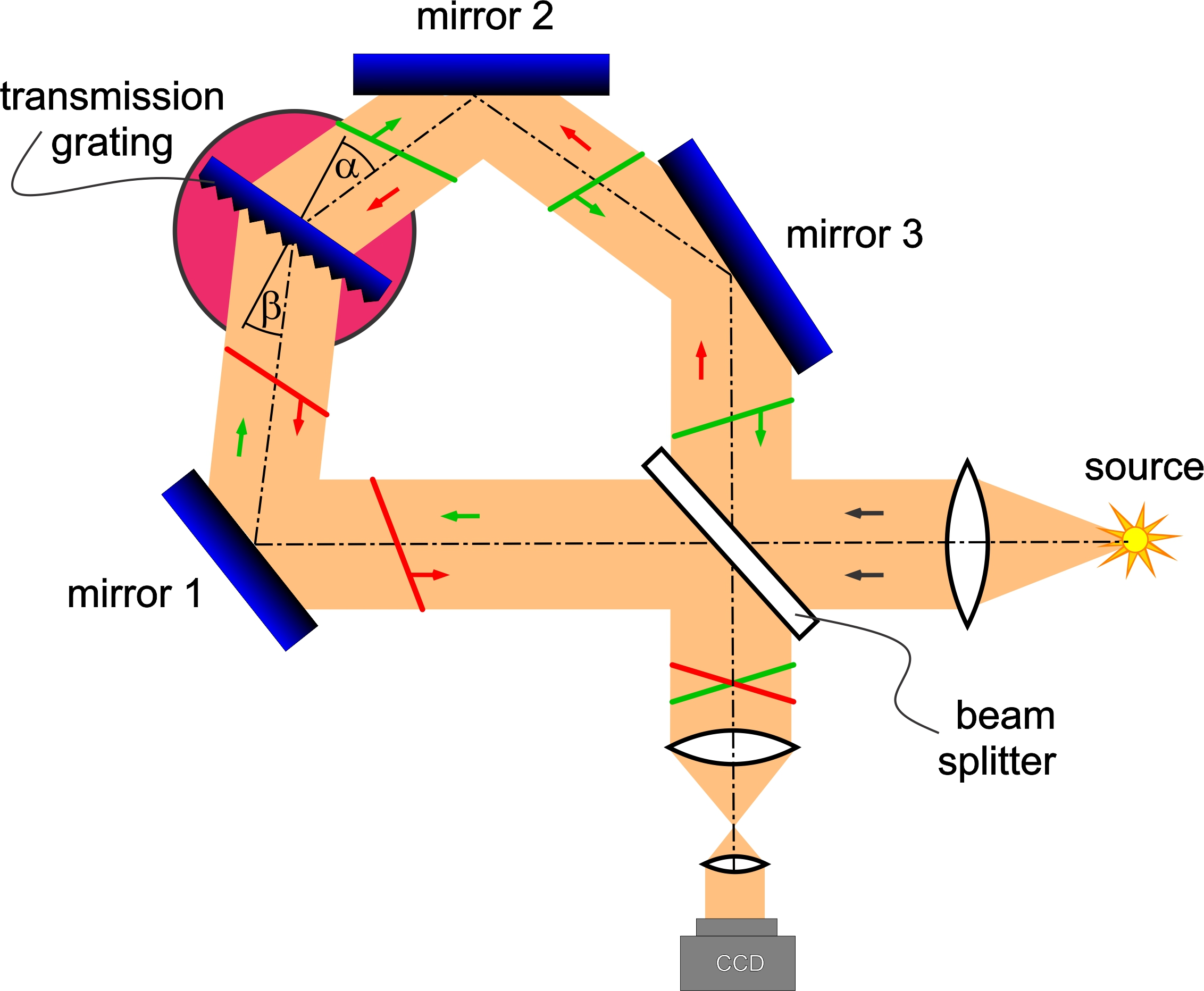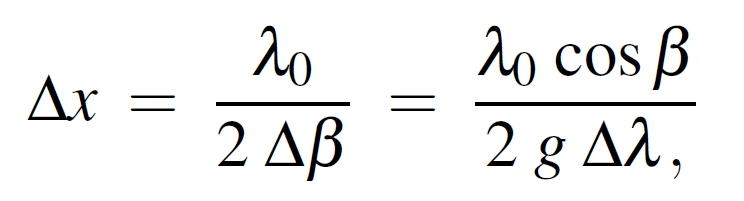The Sagnac Fourier SpectrometerBasically, we are looking at a Sagnac interferometer where one of the mirrors is replaced by a transmission grating. Since the action of a transmission grating is reversible, both directions experience the same diffraction under the same angles at a given wavelength λ0. At this wavelength, the setup works like a standard Sagnac interferometer. For a single wavelength (with zero bandwidth) and the device at rest, there is a homogeneously illuminated field in the output arm (actually dark, since they are exactly 180° out of phase and therefore interfere destructively). For wavelengths off this design wavelength however, the propagation direction (k-vector) is under a small angle to the optical axis, resulting in a tilted wavefront. When these tilted wavefronts interfere in the output arm of the spectrometer, they lead to Fizeau fringes. An odd(!) number of mirrors causes the two wavefronts in the output arm to be oppositely tilted and is therefore indispensible.

The sign convention for transmission gratings is that angles on the same side of the grating normal have the same sign on both sides of the grating. Hence, in the figure above, both angles α and β are positive WLOG. For the wavelength λ0+Δλ, the spacing Δx of these fringes is:where g is the groove density of the transmission grating. The wavelength λ0+Δλ is diffracted under the angle β, the angular dispersion is Δβ/Δλ = g/cosβ. That means, the angular dispersion (and the resolving power of the instrument) increases with the angle β, obviously only limited by the clear aperture.

References:

  Matthias Lenzner and Jean-Claude Diels "A Sagnac Fourier Spectrometer" Opt. Express, 25 (2017) A447-  A453

  Matthias Lenzner
"Sagnac Fourier Spectrometer (SAFOS)" US Patent 10,571,442 ; issued on Feb 25, 2020